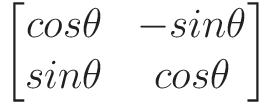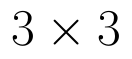Select Page

# Maths 12 Science CBSE Probability Answers for MCQ in English

Maths 12 Science CBSE Probability Answers for MCQ in English to enable students to get Answers in a narrative video format for the specific question.

Expert Teacher provides Maths 12 Science CBSE Probability Answers for MCQ through Video Answers in English language. This video solution will be useful for students to understand how to write an answer in exam in order to score more marks. This teacher uses a narrative style for a question from Probability not only to explain the proper method of answering question, but deriving right answer too.

Please find the question below and view the Answer in a narrative video format.

Question:

## Similar Questions from CBSE, 12th Science, Maths, Probability

Question 1 : Consider the experiment of tossing a coin. If the coin shows head, toss is done again, but if it shows tail, then throw a die. Find the conditional probability of the events that ' the die shows a number greater than 4', given that ' there is atleast one tail'.    (View Answer Video)

Question 2 : Three cards are drawn without replacement from a pack of 52 cards. Find the probability that the cards drawn are king, queen and jack respectively.

Question 3 : A couple has 2 children. Find the probability that both are boys, if it is known that one of them is a boy.  (View Answer Video)

Question 4 : Find the mean, the variance and the standard deviation of the number of doublets in three throws of a pair of dice.  (View Answer Video)

Question 5 : Three cards are drawn without replacement from a pack of 52 cards. Find the probability that the cards drawn are king, queen and jack.

### Determinant

Question 1 : If area of a triangle is 35 sq unit with vertices (2, -6), (5, 4) and (k, 4), then k is, (View Answer Video)

Question 3 : Evaluate  the determinants in :.(View Answer Video)

Question 4 : Let A be a non-singular square matrix of order 3 * 3. Then | adj A | is equal to: (View Answer Video)

Question 5 : Let A be the non- singular square matrix of order, then |adj A| is equal to, (View Answer Video)

### Vector Algebra

Question 1 : Find the unit vector in the direction of vector PQ, where P and Q are the points (1, 2, 3) and (4, 5, 6) respectively. (View Answer Video)

Question 2 : Find the direction cosines of the vector joining the points A(1, 2, -3) and B(-1, -2, 1), directed from A to B. (View Answer Video)

Question 3 :  Find the sum of the vectors :(View Answer Video)

Question 4 : For given vectors,andfind the unit vector in the direction of the vector a + b. (View Answer Video)

Question 5 : Find the unit vector in the direction ofifand(View Answer Video)

### Continuity and Differentiability

Question 1 : Findfor the function. (View Answer Video)

Question 2 : Differentiate w.r.t.x the function, for some fixed a > 0 and x > 0. (View Answer Video)

Question 3 : Differentiate w.r.t.x the function, for some constant a and b. (View Answer Video)

Question 4 : Findfor the function. (View Answer Video)

Question 5 : Differentiate the functionwith respect to x. (View Answer Video)